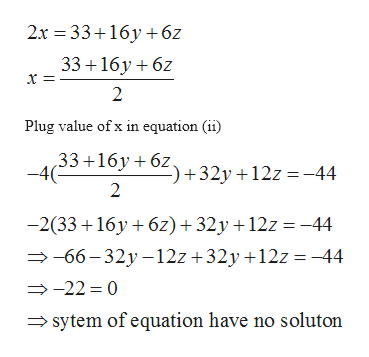# Solve the following system of equations. (Enter your answers as a comma-separated list. If there are infinitely many solutions, enter a parametric solution using t and/or s. If there is no solution, enter NONE.)2x - 16y - 6z= 33-4x + 32y +12z = -44 (x, y, z) =

Question

Solve the following system of equations. (Enter your answers as a comma-separated list. If there are infinitely many solutions, enter a parametric solution using t and/or s. If there is no solution, enter NONE.)

2x - 16y - 6z= 33
-4x + 32y +12z = -44

(x, y, z) =

check_circle

Step 1

Given

2x - 16y - 6z= 33……………..……….(i)

-4x + 32y +12z = -44…………………(ii)

Step 2

From equatio...help_outlineImage Transcriptionclose2x 33 16 6z 33 16y 6 x = 2 Plug value of x in equation (ii) 33+16y 6Z 32y +12z =-44 -4( 2 -2(33 16y 6z)32y + 12z =-44 -66-32y -12z +32y +12z-44 -22 0 sytem of equation have no soluton fullscreen

### Want to see the full answer?

See Solution

#### Want to see this answer and more?

Solutions are written by subject experts who are available 24/7. Questions are typically answered within 1 hour.*

See Solution
*Response times may vary by subject and question.
Tagged in

### Algebra